login  home  contents  what's new  discussion  bug reports help  links  subscribe  changes  refresh  edit

# Edit detail for SandBoxDefineInteger revision 11 of 11

 1 2 3 4 5 6 7 8 9 10 11 Editor: test1 Time: 2013/04/26 18:59:47 GMT+0 Note:

changed:
-    Rep == Record(neg:T, pos:T)
Rep ==> Record(neg:T, pos:T)
rep x ==> (x@%) pretend Rep
per x ==> (x@Rep) pretend %


Why are the domains PositiveInteger? and NonNegativeInteger? defined as SubDomains of Integer insteadof the other way around? Here is a (still somewhat imperfect) example of one way of defining Integer from a more primitive domain (some domain without negatives and perhaps without zero, sometimes called a rig - ring without negative) although Axiom does not currently implement such a category. (Related: category RNG - ring without identity).

John Baez wrote:
Rigs are neglected in ordinary algebra texts, a deficiency that someday must be fixed. Why? First, a lot of stuff that’s true about rings is still true about rigs. Second, and much more importantly, any approach to algebra that doesn’t make room for the natural numbers is clearly defective: the natural numbers are a fundamental algebraic structure that must be reckoned with!

http://golem.ph.utexas.edu/category/2008/05/theorems_into_coffee_iii.html

(Refer also to his discussion of PROPS.)

Most CAS doesn't bother to define natural numbers via the Peano Axioms and then derive integers, for example, as an equivalence class of pairs of natural numbers so that

(a, b) ~ (c, d) iff a + d = b + c.

The question is whether a compiler could be smart enough to replace the definition via equivalence classes by an efficient representation that is, for example, used by GMP.

First let's define a constructor name Difference. Like Fraction the representation will be pairs of some suitable type and equality will be defined by an equivalence relation on this representation. Integer then can be constructed as Difference(CardinalNumber) as a kind of algebraic "quotient".

)abbrev domain DIFF Difference
Difference(T:Join(Monoid,AbelianMonoid,OrderedSet,RetractableTo(NonNegativeInteger))): Join(Monoid,AbelianMonoid, OrderedSet,RetractableTo(NonNegativeInteger)) with
_-:(%,%) -> %
Rep ==> Record(neg:T, pos:T)
rep x ==> (x@%) pretend Rep
per x ==> (x@Rep) pretend %
0 == per [0,0]
1 == per [0,1]
-- binary search for the canonical representative
canonize(x:Rep):Rep ==
y:= [0,0]@Rep
while x.neg+y.pos < x.pos repeat
n:T:=1
while x.neg+y.pos+(nn:=n+n) <= x.pos repeat n:=nn
y.pos:=y.pos+n
while x.neg > x.pos+y.neg repeat
n:T:=1
while x.neg >= x.pos+y.neg+(nn:=n+n) repeat n:=nn
y.neg := y.neg+n
y
x+y == per canonize [rep(x).neg+rep(y).neg, rep(x).pos+rep(y).pos]
x-y == per canonize [rep(x).neg+rep(y).pos, rep(x).pos+rep(y).neg]
x=y == rep(x).pos+rep(y).neg = rep(x).neg+rep(y).pos
x<y == rep(x).pos+rep(y).neg < rep(x).neg+rep(y).pos
x>y == rep(x).pos+rep(y).neg > rep(x).neg+rep(y).pos
-- how to define this properly?
coerce(x:%):OutputForm ==
x<0 => -(rep(x).neg::OutputForm)
rep(x).pos::OutputForm
coerce(x:NonNegativeInteger):% == per [0,coerce(x)$T] spad  Compiling FriCAS source code from file /var/lib/zope2.10/instance/axiom-wiki/var/LatexWiki/7646994448072444383-25px001.spad using old system compiler. DIFF abbreviates domain Difference ------------------------------------------------------------------------ initializing NRLIB DIFF for Difference compiling into NRLIB DIFF ****** Domain: T$ already in scope
****** Domain: T$already in scope ****** Domain: T$ already in scope
processing macro definition Rep ==> Record(neg: T$,pos: T$)
processing macro definition rep x ==> pretend(@(x,$),Record(neg: T$,pos: T$)) processing macro definition per x ==> pretend(@(x,Record(neg: T$,pos: T$)),$)
compiling exported Zero : () -> $Time: 0 SEC. compiling exported One : () ->$
Time: 0 SEC.
compiling local canonize : Record(neg: T$,pos: T$) -> Record(neg: T$,pos: T$)
Time: 0 SEC.
compiling exported + : ($,$) -> $Time: 0 SEC. compiling exported - : ($,$) ->$
Time: 0 SEC.
compiling exported = : ($,$) -> Boolean
Time: 0 SEC.
compiling exported < : ($,$) -> Boolean
Time: 0.01 SEC.
compiling exported > : ($,$) -> Boolean
Time: 0 SEC.
compiling exported coerce : $-> OutputForm Time: 0 SEC. compiling exported coerce : NonNegativeInteger ->$
Time: 0 SEC.
(time taken in buildFunctor:  0)
;;;     ***       |Difference| REDEFINED
;;;     ***       |Difference| REDEFINED
Time: 0 SEC.
Warnings:
 canonize:  neg has no value
 canonize:  pos has no value
 =:  pos has no value
 <:  pos has no value
 >:  pos has no value
 coerce:  neg has no value
 coerce:  pos has no value
Cumulative Statistics for Constructor Difference
Time: 0.01 seconds
finalizing NRLIB DIFF
Processing Difference for Browser database:
--->-->Difference(constructor): Not documented!!!!
--->-->Difference((- (% % %))): Not documented!!!!
--->-->Difference(): Missing Description
; compiling file "/var/aw/var/LatexWiki/DIFF.NRLIB/DIFF.lsp" (written 04 APR 2022 07:06:23 PM):
; /var/aw/var/LatexWiki/DIFF.NRLIB/DIFF.fasl written
; compilation finished in 0:00:00.037
------------------------------------------------------------------------
Difference is now explicitly exposed in frame initial
Difference will be automatically loaded when needed from
/var/aw/var/LatexWiki/DIFF.NRLIB/DIFF

Now use DIFF to define an "Integer"

fricas
i:DIFF(CardinalNumber)
Type: Void
fricas
i:=2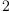(1)
Type: Difference(CardinalNumber?)
fricas
j:=i-4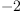(2)
Type: Difference(CardinalNumber?)
fricas
k:=j+2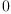(3)
Type: Difference(CardinalNumber?)
fricas
test(k=0)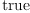(4)
Type: Boolean
fricas
i:=1234567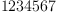(5)
Type: Difference(CardinalNumber?)
fricas
j:=i-7654321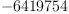(6)
Type: Difference(CardinalNumber?)
fricas
k:=j+6666666-246912(7)
Type: Difference(CardinalNumber?)
fricas
test(k=0)(8)
Type: Boolean

The point of this construction is to illustrate several problems.

One such problem is that current generation of compilers in computer algebra systems such as Axiom, specifically Spad and Aldor, are not able to automatically convert this presumably mathematically correct specification to an efficient implementation, e.g. signed integers.

Another issue is why these languages do not have some built-in support for such common algebraic constructions as "taking a quotient". In particular, there seems to be no general way of defining a "canonical representation".

A mathematician, a biologist and a physicist --Bill Page, Sun, 27 Jul 2008 11:11:48 -0700 reply
are sitting in a street café watching people entering and leaving the house on the other side of the street. First they see two people entering the house. Time passes. After a while they notice three people leaving the house. The physicist says, "The measurement wasn't accurate." The biologist says, "They must have reproduced." The mathematician says, "If one more person enters the house then it will be empty."

http://en.wikipedia.org/wiki/Mathematical_joke